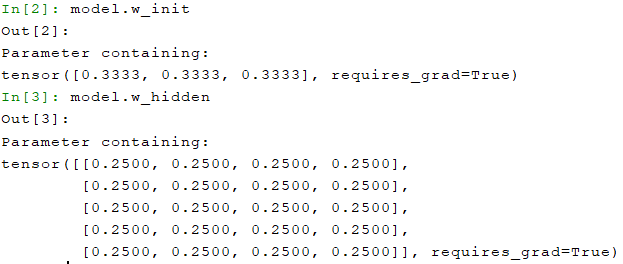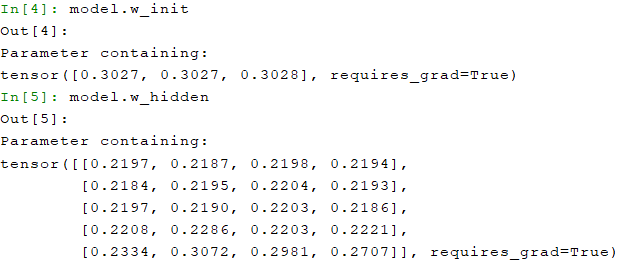I’m trying to apply a sensor-fusion architecture, explained in Vielzeuf, V. et al. - this paper - , to my own problem. The way it works is it takes M separate 1D inputs, each of which enter M twin CNNs. Then, there is an additional twin (M + 1)-nth “CentralNet” connected to the remaining CNNs in such a way that its layers are a linear combination with learnable weights of itself with the corresponding layers of the other twins, as the following diagram suggests.

Where M stands for the modality with numbered by its superscript, C stands for central (CentralNet) and the subscripts denote the number of the layer (Vielzeuf, V. et al.)

I’ve implemented this in PyTorch with the following Model Class:

``````class Model(nn.Module):
def __init__(self, n_mods, conv_layers, conn_layers, kernels, paddings, pools, drop):
super().__init__()

# Number of modalities
self.n_mods = n_mods

# Number of convolutional and fully connected layers
self.conv_len = len(conv_layers) - 1
self.conn_len = len(conn_layers) - 1

# CentralNet input, feature and output linear transformations' weights (uniformly distributed)
self.w_init = nn.Parameter(torch.ones(n_mods) * 1 / n_mods, requires_grad=True)

w_hidden = torch.ones((self.conv_len + self.conn_len), n_mods + 1) * 1 / (n_mods + 1)

#  Build n_mods copies of the required architecture and append to final fusion_net
self.fusion_net = nn.ModuleList()
for mod in range(n_mods + 1):
mod_net = nn.ModuleList()

for D_in, D_out, kernel, padding, pool in zip(conv_layers, conv_layers[1:], kernels, paddings, pools):

for i, D_in, D_out in zip(range(self.conn_len), conn_layers, conn_layers[1:]):
mod_net.append(self.conn_block(D_in, D_out, drop, (i + 1) == self.conn_len))

self.fusion_net.append(mod_net)

def forward(self, x):
# Input arrives as list of n_mods (batch_size, 1, signal_len) tensors
y = list(x)

# Create virtual input for CentralNet with linear combination of other n_mods inputs - mod = 0 is CentralNet
for i in range(self.n_mods):
y += self.w_init[i] * x[i]

# Iterate through each set of corresponding layers from all the n_mods + 1 networks
for block in range(self.conv_len + self.conn_len):
for mod in range(self.n_mods + 1):
for layer in self.get_block(mod, block):
y[mod] = layer(y[mod])

# Perform weighted sum in every set of layers
if mod == 0:
y = y.clone() * self.w_hidden[block, mod]
else:
y = y.clone() + self.w_hidden[block, mod] * y[mod]

# Flatten last set of convolutional layers
if block + 1 == self.conv_len:
y = self.flatten(y)

return y

# Repeating convolutional blocks
def conv_block(self, D_in, D_out, kernel, padding, pool):
conv_module = nn.ModuleList()
conv_module.append(nn.MaxPool1d(pool, stride=2))
conv_module.append(nn.ReLU(inplace=False))
conv_module.append(torch.nn.BatchNorm1d(D_out))
return conv_module

# Repeating fully-connected blocks
def conn_block(self, D_in, D_out, drop, last):
conn_module = nn.ModuleList()
conn_module.append(nn.Linear(D_in, D_out, bias=False))
conn_module.append(nn.ReLU(inplace=False))

# Don't use dropout in last fully-connected layer
if not last:
conn_module.append(nn.Dropout(p=drop))
return conn_module

def flatten(self, y):
N, _, _ = y.size()
for i in range(len(y)):
y[i] = y[i].view(N, -1)
return y

# Get operations from layer block and modality mod
def get_block(self, mod, block):
return list(list(self.fusion_net.children())[mod].children())[block]
``````

Where `n_mods` is an integer for the number of input modalities, `conv_net` a list with the depth size of each convolutional layer (starting with 1), `conn_net` a list with the number of nodes in each fully connected layer (starting with the flattened length number and ending with 1), `kernels` is a list with the kernel size for each convolution, similarly, `padding` is a list with the paddings, `pools` is a list with the pooling size of for each convolutional block and `drop` is a float indicating the dropout probability.

My struggle is that even though the architecture is able to learn as a whole - converge loss and reach high accuracy - the weighted sum parameters barely change from the initial values. The original paper reports how these weights significantly change, giving more importance to some modalities at different stages of the network, as shown:(Vielzeuf, V. et al.)

I’m using the sum of BCEWithLogitsLoss for each `n_mods + 1` modalities’ output as loss function, SGD, L1 normalization for weighted sum parameters, L2 for every other and the same learning rate for both groups (using 0.1 or 0.01 for weighted sum parameters drives them to near 0). Did I not implement it correctly with the “non-conventional” additional learnable parameters and the weighted sums?

i didn’t understand how you are visualizing or noticing all the weights changes?

I call `model.w_init` and `model.w_hidden` before and after an epoch or more and realise the initial values aren’t too far off from the lastly updated ones and very similar amongst each other. For example, with a learning rate of 0.001, starts at:and after one epoch:With a learning rate of 0.01, ends at:It seems like they do change a bit…?

They either fall to very close to zero or change very little, mostly all in the same direction. This would mean they mostly bear the same importance - not much at all. I’m not sure if it is a result that makes sense, hence thinking there could be some implementation problem. Do you also think the implementation is ok? Then I could be worrying needlessly and the weights are actually evolving correctly

The weight changes in individual weight values have to be very small IMO. As long as the model is training and loss is going down, i wont worry.